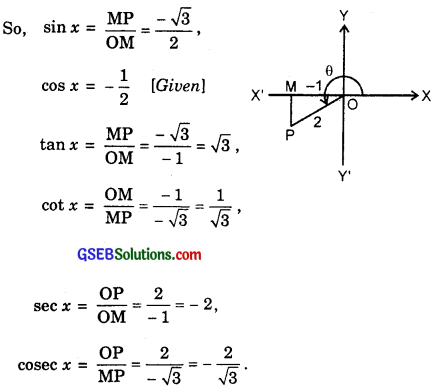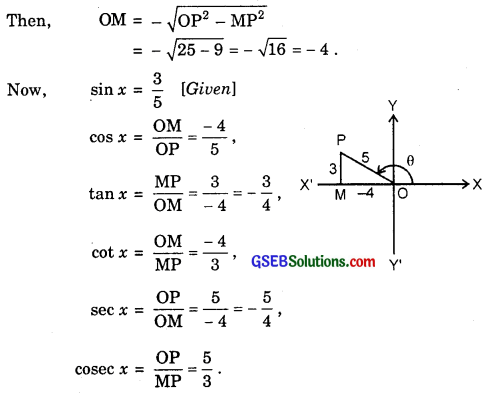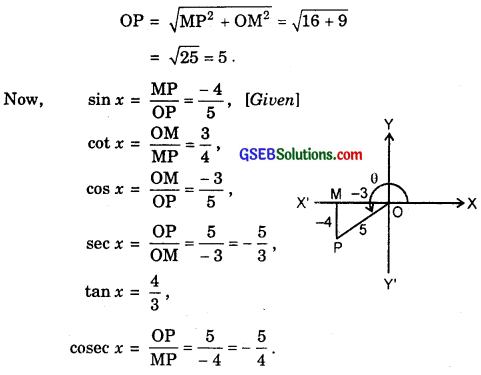# GSEB Solutions Class 11 Maths Chapter 3 Trigonometric Functions Ex 3.2

Gujarat Board GSEB Textbook Solutions Class 11 Maths Chapter 3 Trigonometric Functions Ex 3.2 Textbook Questions and Answers.

## Gujarat Board Textbook Solutions Class 11 Maths Chapter 3 Trigonometric Functions Ex 3.2

Find the values of the other five trigonometric functions in questions 1 to 5:
1. cos x = – $$\frac{1}{2}$$, x lies in third quadrant.
2. sin x = $$\frac{3}{5}$$, x lies in second quadrant.
3. cot x = $$\frac{3}{4}$$, x lies in third quadrant.
4. sec x = $$\frac{13}{5}$$, x lies in fourth quardrant.
5. tan x = – $$\frac{5}{12}$$, x lies in the second quadrant.
Solutions to questions 1 – 5:
1. Since x lies in the 3rd quadrant, therefore
cos x = – $$\frac{1}{2}$$ ⇒ $$\frac{OM}{OP}$$ = $$\frac{- 1}{2}$$.
Let OM = – 1 and OP = 2.
∴ MP = $$\sqrt{\mathrm{OP}^{2}-\mathrm{OM}^{2}}$$ = – $$\sqrt{4 – 1}$$ = – $$\sqrt{3}$$2. Since x lies in the second quadrant, therefore
sin x = $$\frac{3}{5}$$ ⇒ $$\frac{MP}{OM}$$ = $$\frac{3}{5}$$
Let MP = 3, S0, OP = 5.3. Since x lies in third quadrant, therefore
cot x = $$\frac{3}{4}$$ = $$\frac{- 3}{- 4}$$
Let MP = – 4. So, OM = – 3. Then,4. Since x lies in fourth quardrant, therefore
sec x = $$\frac{13}{5}$$ ⇒ $$\frac{OP}{OM}$$ = $$\frac{13}{5}$$ [Given]
Let OP = 13. So, OM = 5. Then,5. x lies in the second quadrantQuestions?
Find the values of the following trigonometric ratios:
6. sin 765°
7. cosec (- 1410°)
8. tan $$\frac{19π}{3}$$
9. sin ($$\frac{-11π}{3}$$)
10. cot ($$\frac{-15π}{4}$$)
Solutions to questions 6 – 10:
6. sin 765° = (8 × 90° + 45°)
= sin 45° = $$\frac{1}{\sqrt{2}}$$.

7. cosec (- 1410°) = – cosec 1410° [∵ cosec (-θ) = – cosec θ
= – cosec (16 × 90° – 30°)
= – cosec (- 30)° = – [- cosec 30°]
= cosec 30° = 2.

8. tan $$\frac{19π}{3}$$ = tan (6π + $$\frac{π}{3}$$) = tan $$\frac{π}{3}$$ = $$\sqrt{3}$$.

9. sin ($$\frac{- 11π}{3}$$) = – sin $$\frac{11π}{3}$$ [∵ sin(-θ) = – sin θ]
= – sin(4π – $$\frac{π}{3}$$) = – (- sin $$\frac{π}{3}$$)
= sin $$\frac{π}{3}$$ = $$\frac{\sqrt{3}}{2}$$.

10. cot($$\frac{- 15π}{4}$$) = – cot $$\frac{15π}{4}$$ [∵ cot(-θ) = – cot θ]
= – cot (4π – $$\frac{π}{4}$$) = – cot (- $$\frac{π}{4}$$)
= – (- cot $$\frac{π}{4}$$) = cot $$\frac{π}{4}$$ = 1.